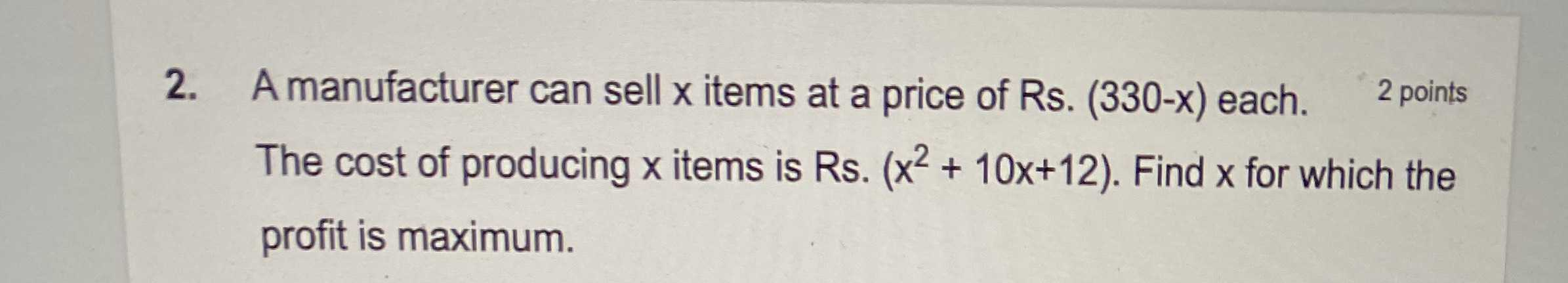### ¿Todavía tienes preguntas de matemáticas?

Pregunte a nuestros tutores expertos
Algebra
PreguntaA manufacturer can sell $$x$$ items at a price of Rs. $$( 330 - x )$$ each.The cost of producing $$x$$ items is Rs. $$( x ^ { 2 } + 10 x + 12 )$$ . Find $$x$$ for which the profit is maximum.